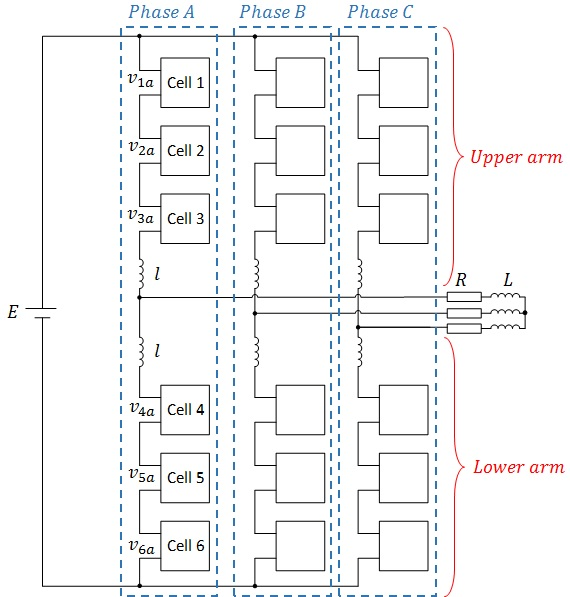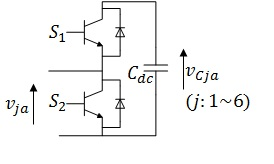LIBRARY

# Sensitivity Analysis of a Modular Multilevel Converter

Year: 2015Fig. 1. (a) Circuit configuration of a modular multilevel inverter: Three phase MMC, and
The modular multilevel converter (MMC), shown in Fig.1, is one of the next generation high/medium-voltage converters intended to achieve a high-power conversion without line-frequency transformers. While the MMC has the advantages of easy assembly and flexible converter design, it still requires a reliable design like any other system. The design criteria in this case are a function of a large number of variables, due to the large number of sub-modules that are usually used in MMCs. Most papers so far have focused on the modeling and control of the MMC, but not on the design. This paper employs a sensitivity analysis technique to develop a detailed switching model to design the most compact power converter possible with the minimum required safety margins.

The many design criteria in an MMC include output power and line-to-line voltage, maximum temperature, peak value of sub module voltage, and power quality. These design criteria are selected based on the intended application. Each of these criteria is a function of a large number of variables, which require equivalent and simplified models. There is a distinction between models used to understand a law and models used to predict the behavior of a system given a presumably understood law. The latter requires more precision; the model used for designing a converter falls within this group.

This paper uses the sensitivity analysis technique to determine the main variables required for consideration in a specific design criteria. The uncertainties associated with these main variables can be propagated through the model via nondeterministic simulations to calculate the required safety margins in the design. Using this technique, one can develop a precise model from a design standpoint, with the minimum number of variables required, in order to have a realistic design without requiring large safety margins.

In the first section, the main concept of the sensitivity analysis is described and the most popular methods are discussed. In the second section, the peak voltage of a power cell and the resonance behavior of a circulating current are selected as the design criteria, which is generally used to select the minimum required capacitance and arm inductance. Sensitivity analysis is performed at three different levels, based on the hierarchical structure of the MMC, in order to develop a model to accurately predict the effects of parametric uncertainties on these design criteria with a minimum number of uncertain variables.Fig. 1. (b) Single power cell